Your browser does not support the IFRAME tag.Home MonkeyNotes Printable Notes Digital Library Study Guides Study Smart Parents Tips College Planning Test Prep Fun Zone Help / FAQ How to Cite New Title Request

 Section 6 : Mathematical Reasoning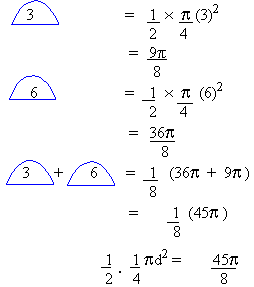d2    =   45                                            = 3Ö 5 Ans : C Let their shares be x and (5000 - x)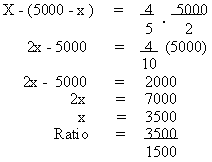= 7 : 3 Ans : A Each interval is of length 2p Let any point in the sixth interval be x. 10p < x < 12p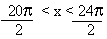Of the choices given, only 23p/2 fits the above condition. Ans : C Formula: Total worth in cents = 10 cents x z coins + 5 cents x (z - 3 coins)           coin                         coin Total worth of coins = 10z + 5 (z - 3)                                = 10z + 5z - 15                                = 15z - 15                                = 15 (z - 1) Ans : C Since the exact mode of intersection is not given, all three options are possible. Ans : C Weight of the heaviest object    :    500 grams Weight of the lightest object      :    25 grams    Ratio      =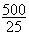=      20 Ans : D (A)  55   ´   55   ´   555                    - 1678875 (B)  5  ´   5   ´   55   ´   555             - 763125 (C)  55   ´   55   ´   55                      - 166375 (D)  5 ´   5   ´   5   ´   5 ´ 5 ´ 5       - 15625     (which is the least) (E)  5 ´   55   ´   555                        - 152625 Ans : D The following integer pairs satisfy the equation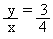(4,3), (8,6), (12,9), (16,12), (20,15), .......and so on. Ans : A             4x + y = 24        If x  =  5  and  y  =  4             4x + y = 24       \ I is satisfied       If x = 2 and y is a multiple of 4            4x + y = 24       \ III is satisfied Ans : C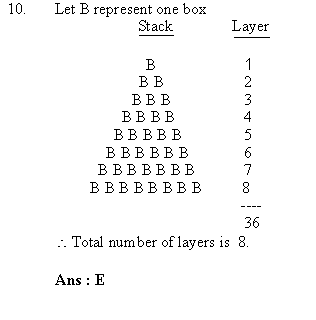Index Part I
 Search: All Products Books Popular Music Classical Music Video DVD Toys & Games Electronics Software Tools & Hardware Outdoor Living Kitchen & Housewares Camera & Photo Cell Phones Keywords: